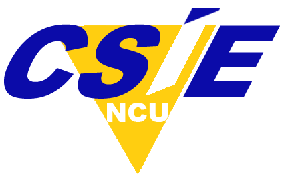# 貝氏資料分析介紹

NCU
Enrollment is Closed## 課程目標

This course provides a bridge between undergraduate training and modern Bayesian methods for data analysis, which is becoming the accepted research standard. Knowledge of algebra and basic calculus is a prerequisite.

## 授課內容

1. Introduction: Credibility, Models, and Parameters
2. The R Programming Language
3. What Is This Stuff Called Probability?
4. Bayes’ Rule
5. Inferring a Binomial Probability via Exact Mathematical Analysis
6. Markov Chain Monte Carlo
7. JAGS
8. Hierarchical Models
9. Model Comparison and Hierarchical Modeling
10. Null Hypothesis Significance Testing
11. Bayesian Approaches to Testing a Point (“Null”) Hypothesis
12. Goals, Power, and Sample Size
13. Stan
14. Overview of the Generalized Linear Model
15. Metric-Predicted Variable on One or Two Groups
1. Course Number

CEA047
2. Classes Start

3. Classes End